# Moment of Inertia Calculation FormulaReading time: 1 minute

## What is Moment of Inertia?

Moment of inertia, also called mass moment of inertia or the angular mass, (SI units kg m2) is a measure of an object's resistance to changes in its rotation rate. It is the rotational analog of mass. That is, it is the inertia of a rigid rotating body with respect to its rotation. The moment of inertia plays much the same role in rotational dynamics as mass does in basic dynamics, determining the relationship between angular momentum and angular velocity, torque and angular acceleration, and several other quantities. While a simple scalar treatment suffices for many situations, a more advanced tensor treatment allows the analysis of such complicated systems as spinning tops and gyroscope motion. The symbol I and sometimes J are usually used to refer to the moment of inertia. The moment of inertia of an object about a given axis describes how difficult it is to change its angular motion about that axis. For example, consider two discs (A and B) of the same mass. Disc A has a larger radius than disc B. Assuming that there is uniform thickness and mass distribution, it requires more effort to accelerate disc A (change its angular velocity) because its mass is distributed further from its axis of rotation: mass that is further out from that axis must, for a given angular velocity, move more quickly than mass closer in. In this case, disc A has a larger moment of inertia than disc B. The moment of inertia has two forms, a scalar form I (used when the axis of rotation is known) and a more general tensor form that does not require knowing the axis of rotation. The scalar form I (often called simply the "moment of inertia") allows a succinct analysis of many simple problems in rotational dynamics, such as objects rolling down inclines and the behavior of pulleys. For instance, while a block of any shape will slide down a friction less decline at the same rate, rolling objects may descend at different rates, depending on their moments of inertia. A hoop will descend more slowly than a solid disk of equal mass and radius because more of its mass is located far from the axis of rotation, and thus needs to move faster if the hoop rolls at the same angular velocity. However, for (more complicated) problems in which the axis of rotation can change, the scalar treatment is inadequate, and the tensor treatment must be used (although shortcuts are possible in special situations). Examples requiring such a treatment include gyroscopes, tops, and even satellites, all objects whose alignment can change. The moment of inertia should not be confused with the polar moment of inertia, which is a measure of an object's ability to resist torsion (twisting).

## Formula of moment of inertia:

A simple formula of the moment of inertia of any object, be it a point mass or a 3D-structure, is given by: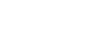where 'dm' is the mass of an infinitesimally small part of the body and r is the (perpendicular) distance of the point mass to the axis of rotation.

## Detailed Analysis

The (scalar) moment of inertia of a point mass rotating about a known axis is defined by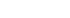The I is additive. Thus, for a rigid body consisting of N point masses mi with distances ri to the rotation axis, the total I equals the sum of the point-mass moments of inertia: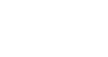For a solid body described by a continuous mass density function ?(r), the I about a known axis can be calculated by integrating the square of the distance (weighted by the mass density) from a point in the body to the rotation axis: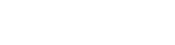where V is the volume occupied by the object. ? is the spatial density function of the object, and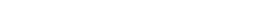are coordinates of a point inside the body.Diagram for the calculation of a disk's I. Here k is 1/2 and r is the radius used in determining the moment. Based on dimensional analysis alone, the I of a non-point object must take the form: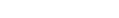where M is the mass R is the radius of the object from the center of mass (in some cases, the length of the object is used instead.) k is a dimensionless constant called the inertia constant that varies with the object in consideration. Inertial constants are used to account for the differences in the placement of the mass from the center of rotation. Examples include: k = 1, thin ring or thin-walled cylinder around its center, k = 2/5, solid sphere around its center k = 1/2, solid cylinder or disk around its center.

## Parallel axis theorem

Once the moment of inertia has been calculated for rotations about the center of mass of a rigid body, one can conveniently recalculate it for all parallel rotation axes as well, without having to resort to the formal definition. If the axis of rotation is displaced by a distance R from the center of mass axis of rotation (e.g. spinning a disc about a point on its periphery, rather than through its center,) the displaced and center-moment of inertia are related as follows: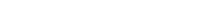This theorem is also known as the parallel axes rule and is a special case of Steiner's parallel-axis theorem.

## Perpendicular Axis Theorem

The perpendicular axis theorem for planar objects can be demonstrated by looking at the contribution to the three axis moments of inertia from an arbitrary mass element. From the point mass moment, the contributions to each of the axis moments of inertia are### Composite bodies

If a body can be decomposed (either physically or conceptually) into several constituent parts, then the moment of inertia of the body about a given axis is obtained by summing the moments of inertia of each constituent part around the same given axis.

## Moments of Inertia of Common Shapes